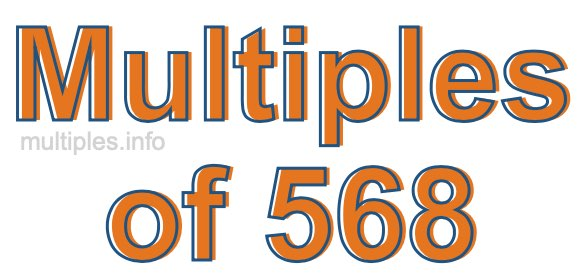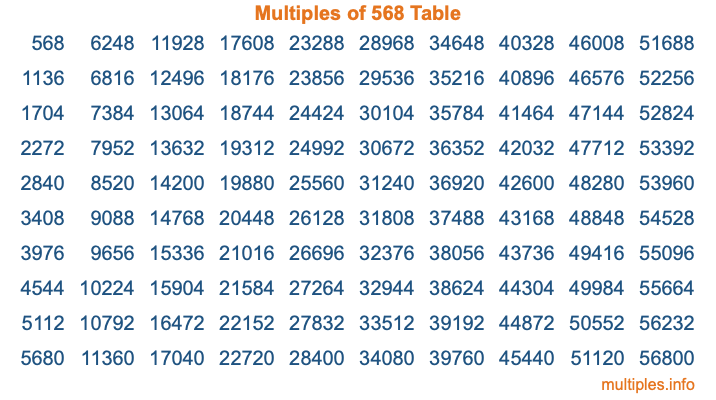Multiples of 568Welcome to the Multiples of 568 page. Here we will first teach you everything you will ever need to know about the multiples of 568, and then give you a study guide summary of everything we taught you to make sure you remember it all. Use this page to look up facts and learn information about the multiples of 568. This page will make you a multiples of five hundred sixty-eight expert!

Definition of Multiples of 568
Multiples of 568 are all the numbers that when divided by 568 equal an integer. Each of the multiples of 568 are called a multiple. A multiple of 568 is created by multiplying 568 by an integer.

Therefore, to create a list of multiples of 568, you start with 1 multiplied by 568, then 2 multiplied by 568, then 3 multiplied by 568, and so on for as long as you want. Thus, the list of the first five multiples of 568 is 568, 1136, 1704, 2272, and 2840. To see a larger list of multiples of 568, see the printable image of Multiples of 568 further down on this page. We also have a category where you can choose any nth multiple of 568.

Multiples of 568 Checker
The Multiples of 568 Checker below checks to see if any number of your choice is a multiple of 568. In other words, it checks to see if there is any number (integer) that when multiplied by 568 will equal your number. To do that, we divide your number by 568. If the the quotient is an integer, then your number is a multiple of 568.

Is  a multiple of 568?

Least Common Multiple of 568 and ...
A Least Common Multiple (LCM) is the lowest multiple that two or more numbers have in common. This is also called the smallest common multiple or lowest common multiple and is useful to know when you are adding our subtracting fractions. Enter one or more numbers below (568 is already entered) to find the LCM.

Check out our LCM Calculator if you need more details about the Least Common Multiple or if you need the LCM for different numbers for adding and subtraction fractions.

nth Multiple of 568
As we stated above, 568 is the first multiple of 568, 1136 is the second multiple of 568, 1704 is the third multiple of 568, and so on. Enter a number below to find the nth multiple of 568.

th multiple of 568

Multiples of 568 vs Factors of 568
568 is a multiple of 568 and a factor of 568, but that is where the similarities end. All postive multiples of 568 are 568 or greater than 568. All positive factors of 568 are 568 or less than 568.

Below is the beginning list of multiples of 568 and the factors of 568 so you can compare:

Multiples of 568: 568, 1136, 1704, 2272, 2840, etc.

Factors of 568: 1, 2, 4, 8, 71, 142, 284, 568

As you can see, the multiples of 568 are all the numbers that you can divide by 568 to get a whole number. The factors of 568, on the other hand, are all the whole numbers that you can multiply by another whole number to get 568.

It's also interesting to note that if a number (x) is a factor of 568, then 568 will also be a multiple of that number (x).

Multiples of 568 vs Divisors of 568
The divisors of 568 are all the integers that 568 can be divided by evenly. Below is a list of the divisors of 568.

Divisors of 568: 1, 2, 4, 8, 71, 142, 284, 568

The interesting thing to note here is that if you take any multiple of 568 and divide it by a divisor of 568, you will see that the quotient is an integer.

Multiples of 568 Table
Below is an image of the first 100 multiples of 568 in a table. The table is in chronological order, column by column. The first column has the first ten multiples of 568, the second column has the next ten multiples of 568, and so on.The Multiples of 568 Table is also referred to as the 568 Times Table or Times Table of 568. You are welcome to print out our table for your studies.

Negative Multiples of 568
Although not often discussed or needed in math, it is worth mentioning that you can make a list of negative multiples of 568 by multiplying 568 by -1, then by -2, then by -3, and so on, to get the following list of negative multiples of 568:

-568, -1136, -1704, -2272, -2840, etc.

Multiples of 568 Summary
Below is a summary of important Multiples of 568 facts that we have discussed on this page. To retain the knowledge on this page, we recommend that you read through the summary and explain to yourself or a study partner why they hold true.

There are an infinite number of multiples of 568.

A multiple of 568 divided by 568 will equal a whole number.

568 divided by a factor of 568 equals a divisor of 568.

The nth multiple of 568 is n times 568.

The largest factor of 568 is equal to the first positive multiple of 568.

568 is a multiple of every factor of 568.

568 is a multiple of 568.

A multiple of 568 divided by a divisor of 568 equals an integer.

568 divided by a divisor of 568 equals a factor of 568.

Any integer times 568 will equal a multiple of 568.

Multiples of a Number
Here you can get the multiples of another number, all with the same attention to detail as we did for multiples of 568 on this page.

Multiples of
Multiples of 569
Did you find our page about multiples of five hundred sixty-eight educational? Do you want more knowledge? Check out the multiples of the next number on our list!